﻿ Project Maths | Geometry
Working together to improve teaching & learning Leagan Gaeilge
Professional Development ›› GeometryWelcome to our Geometry page. We have resources outlining the key Van Hiele Theory of Geometric Understanding along with examples that you can use in the class room. We also have tutorials that will take you through a construction/theorem from the Geometry Course of Study at Junior or Leaving Certificate. The tutorials will explore both conceptual and procedural knowledge  for teaching and learning . Each video concludes with a list of additional resources available on the website for use in the classroom.

### The Van Hiele Theory of Geometric Understanding

This theory explains how students learn Geometry( Trigonometry, Synthetic Geometry, Cooordinate Geometry and Transformational Geometry). An understanding of this theory is key to any Geometry for Understanding classroom.

We have included some links to explanatory videos, diagrams, articles, examples that you can use in your classroom as well as links to previous workshops on the topic and our upcoming workshop

Van Hiele Key Concepts

A two minute introductory video explaining the implications of the 5 Levels in the Van Hiele Theory of Geometric Thought

Van Hiele Example 1 This Sideshow explores teaching 3D Trigonometry with accompanying teachers notes explaining how to incorporate the 5 phases of the learning of the Van Hiele Theory

Van Hiele Example 2 This Sideshow explores teaching the equation of the circle with accompanying teachers notes explaining how to incorporate the 5 phases of the learning of the Van Hiele Theory

A brief article explaining the Van Hiele Theory of Geometric Thought

Previous Workshops on Geometry and the Van Hiele Theory

#### Geometry & Trigonometry Workshop

Project Maths Workshop 2

Upcoming Workshop

### Constructions in Geometry

Bisector of an Angle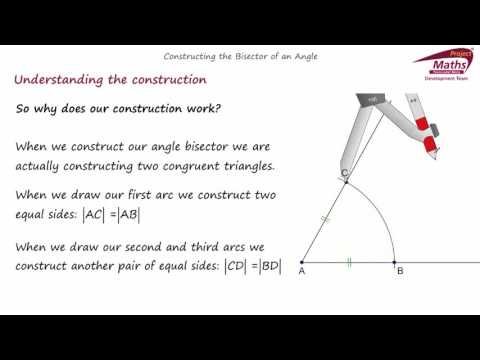How do we construct the bisector of an angle? How does the construction work? How ca...

Perpendicular Bisector of a Line Segment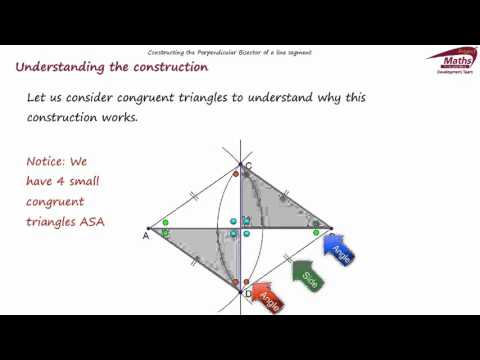How do we construct the perpendicular bisector of a line segment? How does the const...

Circumcentre and Circumcircle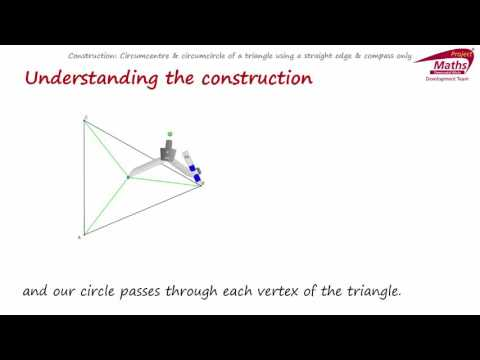How do we construct the circumcircle of a triangle? How does the construction work? ...

Incentre and Incircle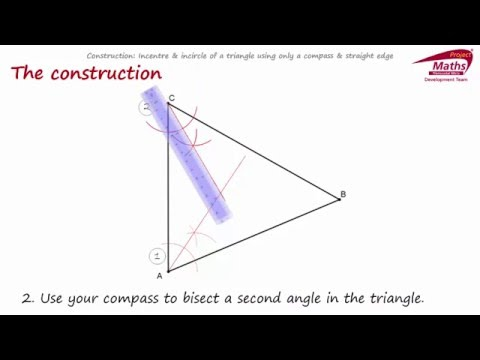How do we construct the incircle of a triangle? How does the construction work? How ...

Angle of 60 degrees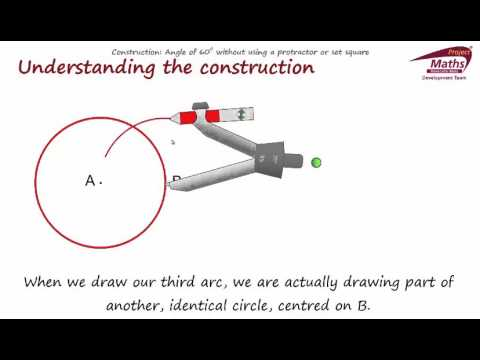How do we construct the an angle of 60 degrees without a protractor or set square? H...

### Theorems in Geometry

Vertically-opposite angles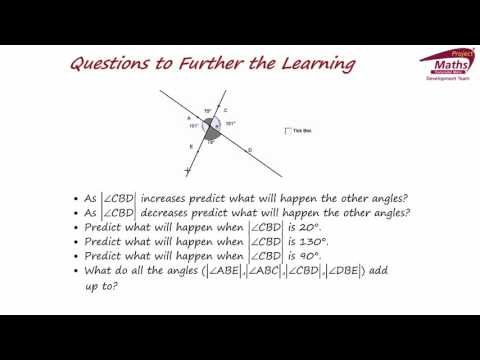Teaching a theorem using discovery learning, algebra as sense making and generalisin...